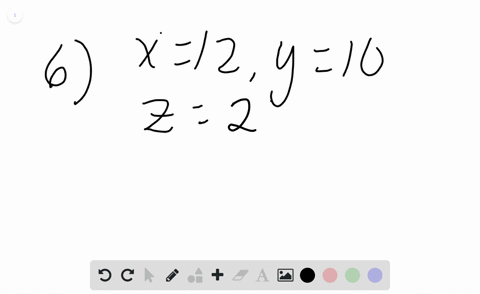Enroll in one of our FREE online STEM bootcamps. Join today and start acing your classes!View Bootcamps### In Exercises $6-14, x=12, y=10,$ and $z=24 .$ Wri…

00:58Problem 5

# $A B C D$ is a parallelogram. Find the value of each ratio. $A D :$ perimeter of $A B C D$

## Discussion

You must be signed in to discuss.

## Video Transcript

we know that a B plus P C plus C d plus d A is gonna be 48 there forever ratio of 9 to 48. They're both divisible by three, giving us 3 to 16.